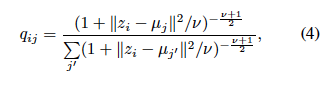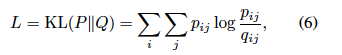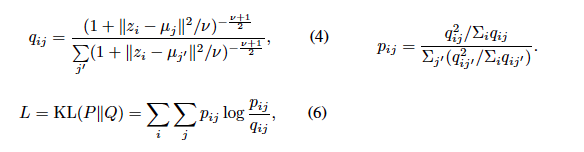# Custom Loss KL-divergence Error

import torch
from common.constants import Constants
import torch.nn.functional as F

class Cluster_Assignment_Hardening_Loss(torch.nn.Module):

def __init__(self):
super(Cluster_Assignment_Hardening_Loss,self).__init__()

def forward(self,encode_output, centroids):

# Calculate q_ij (Eqn-4 of the paper)
q_temp = Variable(torch.zeros(size = (len(encode_output),len(centroids)), requires_grad=True))

for i in range(len(encode_output)):
for j in range(len(centroids)):
a = encode_output[i] - centroids[j]
b = torch.pow(a.norm(2),2)
q_temp[i][j] = torch.pow((1+b/Constants.MU),-(Constants.MU+1)/2)

col_sum = torch.sum(q_temp,dim=1)  # --
for i in range(len(encode_output)):
q_temp[i] = q_temp[i]/col_sum[i]

# Calculate p_ij (Eqn-5 of paper)
p_temp = Variable(torch.zeros(size = (len(encode_output),len(centroids)), requires_grad=True))
temp = torch.pow(p_temp,2)
row_sum = torch.sum(q_temp,dim=0)     # ||

for j in range(len(centroids)):
p_temp[:,j] = q_temp[:,j]/row_sum[j]

col_sum = torch.sum(p_temp, dim=1)  # --
for i in range(len(encode_output)):
p_temp[i] = p_temp[i]/col_sum[i]

kl_div = F.kl_div(p_temp, q_temp)
return kl_div


I have created a custom loss function which ultimately calculates KL-diveregence between p_temp and q_temp. Both the encode_input and centroids are tensors.

But I am getting the following error:
kl_div = F.kl_div(p_temp, q_temp)
RuntimeError: the derivative for ‘target’ is not implemented

What could be the issue?

This is quite literally what it says: F.kl_div does not support taking gradients w.r.t. the second (target) argument and you q_temp asks for gradients (requires_grad=True). If you want the derivative for the second argument as well, you would need to spell out the kl_div, too.

If I may say so, you likely want to replace the for loops over coordinates with clever broadcasting, this is usually much, much faster to the point where it is an error to do for loops over coordinates (very narrow exceptions apply when you would have unreasonably large intermediates or somesuch).

Best regards

Thomas

@tomThis is what I am trying to implement
But what do you mean “spell out”.
How should I handle this case? Can you please explain in detail

Can you suggest a cleaner way to do this?
I will clean the code for broadcasting as you said once it works

Assuming p and q are probability distributions and you want kl_div to be D(p||q) (which is not what F.kl_div does because it has log probabilities on p and I think it does D(q||p)) ),

  kl_div = (p_temp*(p_temp.log()-q_temp.log())).sum()


would give D(p||q). For stability, you could use p_temp.clamp(min=1e-7).log() or somesuch for the and similarly for q. Also, directly computing the log can enhance stability.

Best regards

Thomas

@tom

This is my custom loss class:

import torch
from common.constants import Constants
import torch.nn.functional as F
import torch.nn as nn

class Cluster_Assignment_Hardening_Loss(torch.nn.Module):

def __init__(self):
super(Cluster_Assignment_Hardening_Loss,self).__init__()

def forward(self,encode_output, centroids):

# Calculate q_ij (Eqn-4 of the paper)
q_temp = Variable(torch.zeros(size = (len(encode_output),len(centroids)), requires_grad=True).cuda())

for i in range(len(encode_output)):
for j in range(len(centroids)):
a = encode_output[i] - centroids[j]
b = torch.pow(a.norm(2),2)
q_temp[i][j] = torch.pow((1+b/Constants.MU),-(Constants.MU+1)/2)

col_sum = torch.sum(q_temp,dim=1)  # --
for i in range(len(encode_output)):
q_temp[i] = q_temp[i].clone()/col_sum[i]

# Calculate p_ij (Eqn-5 of paper)
#p_temp = Variable(torch.zeros(size = (len(encode_output),len(centroids)), requires_grad=True).cuda())
temp = torch.pow(p_temp,2)
row_sum = torch.sum(q_temp,dim=0)     # ||

for j in range(len(centroids)):
p_temp[:,j] = q_temp[:,j]/row_sum[j]

col_sum = torch.sum(p_temp, dim=1)  # --
for i in range(len(encode_output)):
p_temp[i] = p_temp[i].clone()/col_sum[i]

kl_div = (p_temp * (p_temp.clamp(min=1e-7).log()  - q_temp.clamp(min=1e-7).log())).sum()
return kl_div


But I am unable to train my network.

I am getting the following error:
File “/usr/prakt/python3.5/site-packages/torch/autograd/init.py”, line 89, in backward
allow_unreachable=True) # allow_unreachable flag
RuntimeError: leaf variable has been moved into the graph interior

Now is a good time to vectorize your codeWhat the error means is that autograd doesn’t like how you assign to bits of your variable (which makes it hard to tell what’s going on if things get overwritten). Most of the need to do so will go away anyway when you write the code better.

p_temp = Variable(q_temp.data.clone(), requires_grad=True).cuda()


this will also trip you, as .cuda() counts as computation and you won’t get a grad on p_temp. If you’re on 0.4, use p_temp = q_temp.clone().detach().cuda().requires_grad_() or so.

Best regards

Thomas

@tom
I have changed the code accordingly, but still getting the same error. Even after broadcasting I am getting the same error

def forward(self,encode_output, centroids):

# Calculate q_ij (Eqn-4 of the paper)
q_temp = Variable(torch.zeros(size=(len(encode_output),len(centroids))).cuda())

for j in range(len(centroids)):
val = encode_output - centroids[j]
nom = torch.pow(val.norm(2,dim=1),2)
q_temp[:,j] = torch.pow((1+nom/Constants.MU),-(Constants.MU+1)/2)

col_sum = torch.sum(q_temp,dim=1)  # --
for i in range(len(encode_output)):
q[i] = q[i].clone()/col_sum[i]

# Calculate p_ij (Eqn-5 of paper)
p_temp = torch.pow(p_temp,2)
row_sum = torch.sum(q,dim=0)     # ||

p_temp /= row_sum

col_sum = torch.sum(p_temp, dim=1)  # --
for i in range(len(encode_output)):
p[i] = p[i].clone()/col_sum[i]

kl_div = (p * (p.clamp(min=1e-7).log()  - q.clamp(min=1e-7).log())).sum()
return kl_div


What am I missing? I could not really understand (I am new to pytorch)

The assignments q[i] =, q_temp[:, j], p[i] = are likely the source of your problem. If you manage to write them as one operation filling everything, it will probably go away.
The snippet you post has too many “free” variables to try to execute it, but it vaguely looks like it should be easy to eliminate the for loops.

Best regards

Thomas

@tom
I tried to eliminate as many loops a I could. And I am not able to think of any way of eliminating these ones that are left.

Can you suggest some way on how can I do this?This is what I am trying to calculate.
($z_i$ is a one-dim tensor(Encoder output) , $\mu_j$ is also a on-dim tensor after doing some manipulations on $$z_i$$ )

Any help is appreciated

I write the dimensions in the comments. Given:

z = torch.randn(7,5)   # i, d use torch.stack([list of z_i], 0) if you don't know how  to get this otherwise.
mu = torch.randn(6,5)  # j, d
nu = 1.2


you do

# I don't use norm. Norm is more memory-efficient, but possibly less numerically stable in backward
q_raw = (1 + ((z.unsqueeze(1)-mu.unsqueeze(0))**2).sum(2) / nu)**(-(nu+1) / 2)  # i, j
q_sum = q_raw.sum(1, keepdim=True) # i, 1 --> will be broadcast
q = q_raw / q_sum # i, j

p_raw = q**2 / q.sum(0, keepdim=True) # i, j
p_sum = p_raw.sum(1, keepdim=True)    # 1, j --> will be broadcast
p = p_raw / p_sum

kl_div = (p * (p.clamp(min=1e-7).log()  - q.clamp(min=1e-7).log())).sum()


Kindly send your invoicing address by PM.Best regards

Thomas

1 Like

@tom

Thanks. It works now

I have one more question to ask.

I want to use this loss combined with MSE error loss to optimize my network

            loss = alpha * loss_mse + (1 - alpha) * loss_kl_div
error += loss.item()
loss.backward()
optimizer.step()


Will this work for what I wish to achieve?

@tom

PM sent.